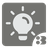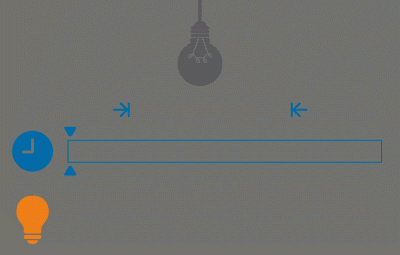Lightbulb End Watching
Simple
English RU

In the third mission from the series about lightbulbs, I want to separately highlight the process and the period of observation of this process.

In the previous mission, the start_watching parameter was introduced, and in this one - the end_watching parameter, which tells the time when it’s necessary to end the observation. If it’s not passed, the mission works as in the previous version, with no observation time limit.

One more update is that the amount of elements (button clicks) can be odd (previously there was a precondition, that the amount of elements is always even).Input: Three arguments and only the first one is required. The first one is a list of datetime objects, the second and the third ones are the datetime objects.

Output: A number of seconds as an integer.

Example:

```sum_light([
datetime(2015, 1, 12, 10, 0, 0),
datetime(2015, 1, 12, 10, 10, 10),
datetime(2015, 1, 12, 11, 0, 0),
],
datetime(2015, 1, 12, 10, 10, 0),
datetime(2015, 1, 12, 11, 0, 10)) == 20

sum_light([
datetime(2015, 1, 12, 10, 0, 0),
],
datetime(2015, 1, 12, 9, 9, 0),
datetime(2015, 1, 12, 10, 0, 0)) == 0

sum_light([
datetime(2015, 1, 12, 10, 0, 0),
datetime(2015, 1, 12, 10, 10, 10),
datetime(2015, 1, 12, 11, 0, 0),
datetime(2015, 1, 12, 11, 10, 10),
],
datetime(2015, 1, 12, 9, 0, 0),
datetime(2015, 1, 12, 10, 5, 0)) == 300
```

Precondition:

• The array of pressing the button is always sorted in ascending order.
• The array of pressing the button has no repeated elements.
• The minimum possible date is 1970-01-01
• The maximum possible date is 9999-12-31